#### Electric Field Model¶

To simulate physical processes such as movement of charged mass particles, particle and liquid dielectrophoresis, and electro-osmosis, an electric field distribution is needed. In FLOW-3D , the electric potential is solved for using the following equation.

(75)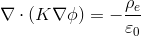with the electric field calculated by

(76)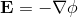where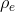,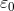are free charge density (i.e., electric charge per unit volume) and permittivity of vacuum or void (defined by elperm in FLOW-3D ) respectively while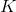is the spatially-varying dielectric constant.

Numerical solution of the Poisson equation Eq. (75) is done by an iterative solver using the GMRES method.

At open boundaries (i.e., boundaries through which fluid flow can occur), either an insulation condition (i.e.,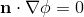where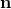is a normal vector on the boundary in question) or a specified value of the electric field can be specified. Solid objects can be assigned time-dependent potentials if they are conductors. Alternatively, an object can be a dielectric material with an assigned dielectric constant. In this case, the electric potential is computed within the object. Also, solid dielectrics may have non-zero conductivities that support free charges and currents.

Optionally, a charge density equation that includes charge convection, charge relaxation and charge sources associated with non-uniform electric properties is solved simultaneously with the electric potential. Please refer to Electric Fields in the Model Reference chapter and the Technical Notes listed at the end of this section for more information.

Most people use the SI system of units for electrostatic and electromagnetic problems. In the SI system the standard unit of charge is the coulomb and the unit of potential is the volt. Electric field intensities are measured in Newton/coulomb in the SI system. When using the CGS system of units, it is customary to express charge and potential in the “electrostatic” units of statvolt and statcoulomb, where:

1 coulomb (C) = 2.998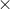109 statcoulomb (statC)

1 volt (V) = 3.33610-3 statvolt (statV)

Electric forces are then,

1 Newton = 1 CVm-1 = 105 dyne

1 dyne = 1 statCstatVcm-1 = 10-5 Newton

If the fluid or solid regions are conducting then charges may develop in response to the applied field and to changes in conductivity and dielectric properties. If a thermal model is activated then Joule heating due to these currents is computed in all conducting fluids and solids. The heat source per unit open cell volume and time associated with passing electric current through conducting fluid,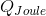, is

(77)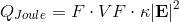where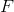and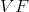are the fluid and open volume fractions in cell and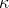is the fluid electrical conductivity. For two-fluid problems,is replaced with 1.0 andwith the volume-averaged mixture value of the electrical conductivity. Similarly, the Joule heat source in solid is

(78)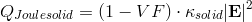More details are given in Flow Science Technical Notes #52, #56, #69 and #70 on the users site at http://users.flow3d.com/technical-notes/.

#### Electro-osmosis¶

Generally, most substances such as silica and glass will acquire a surface electric charge when brought into contact with an aqueous (polar) medium (electrolyte solution), as shown in the figure below.A layer called the electric double layer (EDL) is formed close to the charged surface in which there is an excess of counter-ions over co-ions to neutralize the surface charge. There are more counter-ions than co-ions in the region near the fluid/solid interface. The electric potential created due to the EDL is called the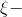potential and is assumed to be imposed at the solid surface. Thepotential is a property of the solid-liquid pair and can be measured experimentally. The thickness of the EDL is indicated by the following parameter, called the Debye shielding distance or Debye length.

(79)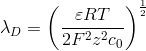where:

•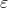= liquid permittivity
•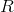= gas constant
•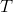= temperature
•= Faraday’s constant
•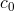= ion concentration
•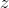= valence

More details on EDL and related physics can be found in [Pro94].

Electro-osmotic flow or electro-osmosis refers to the fluid motion that occurs when an electric field is applied to an electrolyte solution in the vicinity of a charged surface. The process can be described by the following equations

(80)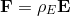(81)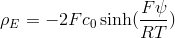(82)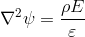where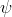is thepotential. An insulation-like boundary condition is imposed for thepotential on all mesh boundaries with a symmetry condition. On solid (obstacle) surfaces, thepotential is imposed. Here Boltzmann charge density distribution for liquid with single valence is assumed. But the user can easily provide other different charge density distributions in two simple functions named psi and dfdpfi released to users. How to do this customization is described in these two functions.

Please refer to Electro-osmosis in Model Reference to get related information on how to use the model.

#### Particle Movement and Fluid Flow Due to Electric Field¶

An electric charge or charge dipoles can be carried by molecules, small droplets, and particles which are called charge, or charge dipole carriers, or mass particles in FLOW-3D . If the charge of a mass particle,, is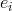, the electric force (called coulomb force) acting on this particle is

(83)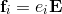where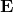is the electric field intensity and its calculation is described above. If a mass particle,, carries charge dipoles and the corresponding dipole moment is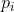, the electric force (called polarization force) imposed on this mass particle is

(84)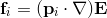If all energy losses in the carriers are neglected, the dipole moment is calculated by

(85)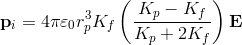where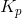is the particle dielectric constant,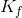is the fluid dielectric constant,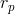is the particle radius and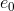is the permittivity of vacuum. Then, the electric force imposed on mass particle,, carrying the charge dipoles can be cast into

(86)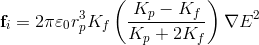Instead of paying attention to the carriers mentioned above, we consider fluid where these charges or charge dipole carriers are distributed. According to Newton’s law, the fluid will experience some body forces due to existence of charge or charge dipoles. The body force due to free charge is

(87)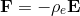whereis the free charge density. The body force due to charge dipoles is

(88)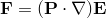where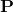is the density of the dipole moment. For dilute dipoles in fluid, this density can be calculated by

(89)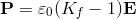The body force is then calculated by

(90)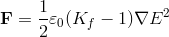In FLOW-3D , users can provide a charge density distribution. This distributed charge density is defined using the FLOW-3D scalar variable having index IECHRG (a FLOW-3D input parameter).

Movement of particles carrying induced charge dipoles due to polarization is called particle Dielectrophoresis (DEP) while fluid flow due to existence of charge dipoles from polarization is called liquid Dielectrophoresis.

With the aforementioned equations included in FLOW-3D , users can simulate both particle and liquid Dielectrophoresis. Please refer to Dielectrophoresis to get related information on how to use the models.